# GMAT Math : DSQ: Calculating the area of a circle

## Example Questions

### Example Question #1 : Circles

You are given a circle and a square. Which one has the larger area?

Statement 1: The radius of the circle is two-thirds the sidelength of the square.

Statement 2: The circumference of the circle is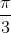times the perimeter of the square.

BOTH statements TOGETHER are insufficient to answer the question.

EITHER statement ALONE is sufficient to answer the question.

BOTH statements TOGETHER are sufficient to answer the question, but NEITHER statement ALONE is sufficient to answer the question.

Statement 2 ALONE is sufficient to answer the question, but Statement 1 ALONE is NOT sufficient to answer the question

Statement 1 ALONE is sufficient to answer the question, but Statement 2 ALONE is NOT sufficient to answer the question.

EITHER statement ALONE is sufficient to answer the question.

Explanation:

Letbe the sidelength of the square. Then its area is.

From Statement 1, it follows that the radius of the circle is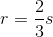From Statement 2,  it follows that , since the perimeter of the square is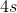, the circumference of the circle is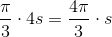, and the radius is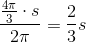- the same fact given in Statement 1.

Either way, it follows that the area of the circle in terms ofis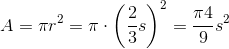,

so all we have to do is compare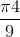to 1 in order to determine whether the square or the circle is larger in area.

### Example Question #1 : Dsq: Calculating The Area Of A Circle

You are given a circle and an equilateral triangle. Which one has the greater area?

Statement 1: The sidelength of the triangle is three times the radius of the circle.

Statement 2: The perimeter of the triangle is 99 inches.

Statement 1 ALONE is sufficient to answer the question, but Statement 2 ALONE is NOT sufficient to answer the question.

EITHER statement ALONE is sufficient to answer the question.

BOTH statements TOGETHER are sufficient to answer the question, but NEITHER statement ALONE is sufficient to answer the question.

Statement 2 ALONE is sufficient to answer the question, but Statement 1 ALONE is NOT sufficient to answer the question.

BOTH statements TOGETHER are insufficient to answer the question.

Statement 1 ALONE is sufficient to answer the question, but Statement 2 ALONE is NOT sufficient to answer the question.

Explanation:

From Statement 2, we can calculate the area of the triangle, but we are given no clues about the area of the circle, actual or relative.

From Statement 1, we know that if we call the radius of the circle, we know the sidelength of the triangle is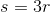.

The area of the circle is.

The area of the triangle is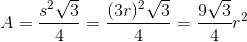.

All we have to do is compareto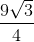to determine whether the circle or the triangle has the greater area.

### Example Question #3 : Circles

Data Sufficiency Question

Calculate the area of a circle.

1. The radius of the circle is 4.

2. The circumference of the circle is 24.

Statement 2 alone is sufficient, but statement 1 alone is not sufficient to answer the question

Both statements taken together are sufficient to answer the question, but neither statement alone is sufficient

Statements 1 and 2 together are not sufficient, and additional data is needed to answer the question

Statement 1 alone is sufficient, but statement 2 alone is not sufficient to answer the question

Each statement alone is sufficient

Each statement alone is sufficient

Explanation:

The area of a circle can be calcuated using the equation: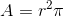and the circumference calculated using: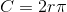The radius is the only information required for calculating the area of a circle and that can be obtained from the circumference, therefore, either statement is sufficient.

### Example Question #4 : Circles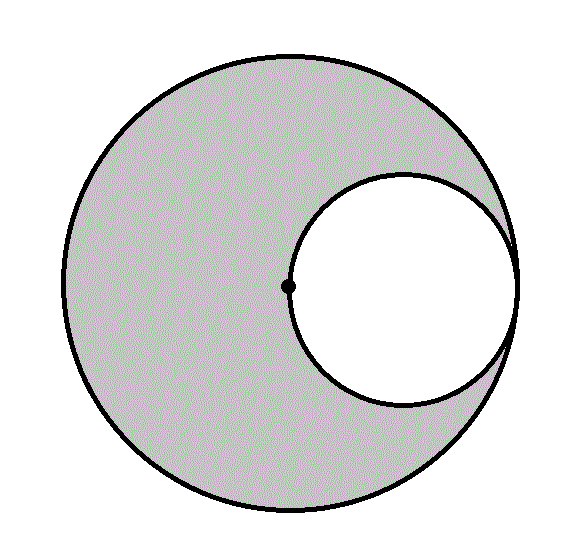What is the area of the gray region in the above figure?

Statement 1: The diameter of the larger circle is one mile.

Statement 2: The radius of the smaller circle is 1,320 feet.

BOTH statements TOGETHER are sufficient to answer the question, but NEITHER statement ALONE is sufficient to answer the question.

BOTH statements TOGETHER are insufficient to answer the question.

Statement 2 ALONE is sufficient to answer the question, but Statement 1 ALONE is NOT sufficient to answer the question.

Statement 1 ALONE is sufficient to answer the question, but Statement 2 ALONE is NOT sufficient to answer the question.

EITHER statement ALONE is sufficient to answer the question.

EITHER statement ALONE is sufficient to answer the question.

Explanation:

The radius of the larger circle is equal to the diameter of the smaller, and, subsequently, twice the radius of the smaller. If one radius is known, then the other can be calculated, and the areas of the two circles can be as well; the difference between their areas is the area of the gray region. Statement 1 tells us the diameter of the large circle, from which its radius can be determined by dividing by 2; Statement 2 tells us the radius of the radius of the smaller circle. From either, the radius of the other circle can be calculated.

### Example Question #5 : Circles

Two circles are constructed; one is inscribed inside a given regular hexagon, and the other is circumscribed about the same hexagon.

What is the area of the inscribed circle?

Statement 1: The area of the circumscribed circle is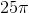Statement 2: The perimeter of the hexagon is 30.

BOTH statements TOGETHER are insufficient to answer the question.

BOTH statements TOGETHER are sufficient to answer the question, but NEITHER statement ALONE is sufficient to answer the question.

Statement 1 ALONE is sufficient to answer the question, but Statement 2 ALONE is NOT sufficient to answer the question.

EITHER statement ALONE is sufficient to answer the question.

Statement 2 ALONE is sufficient to answer the question, but Statement 1 ALONE is NOT sufficient to answer the question.

EITHER statement ALONE is sufficient to answer the question.

Explanation:

Examine the diagram below, which shows the hexagon, segments from its center to two vertices and the midpoint of a side, and the two circles.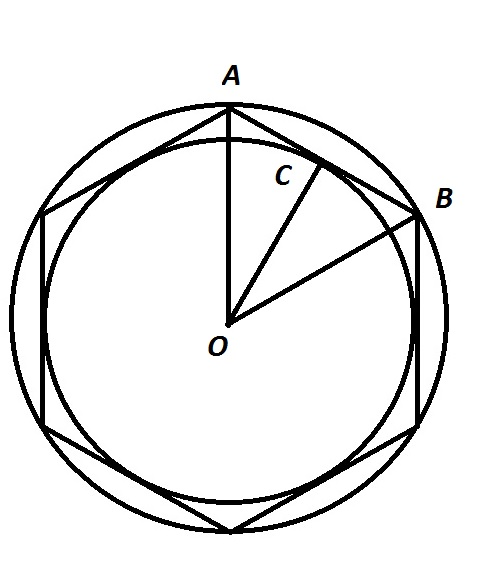From Statement 1 alone, the radius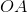of the circumscribed circle can be calculated using the area formula. From Statement 2 alone, the perimeter can be divided by 6 to obtain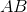; since two consecutive radii and one side of a hexagon can be proved to form an equilateral triangle,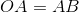. As a consequence, from either statement alone,can be calculated.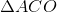can be proved a 30-60-90 triangle, so the 30-60-90 Theorem can be used to calculate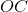, the radius of the inscribed circle. From this, the area of the inscribed circle can be calculated.

Tired of practice problems?

Try live online GMAT prep today.